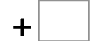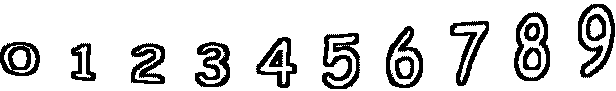5= 10   0= 110= 10   5= 10   10= 16   7= 15 0= 6   1= 9   6= 7   10= 15